# 使用OpenCV和Python构建自己的车辆检测模型

### 概述

• 你对智慧城市的想法感到兴奋吗？如果是的话，你会喜欢这个关于建立你自己的车辆检测系统的教程的

• 在深入实现部分之前，我们将首先了解如何检测视频中的移动目标

• 我们将使用OpenCV和Python构建自动车辆检测器

### 介绍

• 白天交通路口有多少辆车？
• 什么时候交通堵塞？
• 什么样的车辆（重型车辆、汽车等）正在通过交叉路口？
• 有没有办法优化交通，并通过不同的街道进行分配？

https://youtu.be/C_iZ2yivskE

### 目录

1. 视频中运动目标检测的思想

2. 视频中目标检测的真实世界用例

3. 视频目标检测的基本概念

• 帧差分

• 图像阈值

• 检测轮廓

• 图像膨胀

4. 利用OpenCV构建车辆检测系统

#### 视频中运动目标检测的思想#### 视频目标检测的基本概念##### 帧差分##### 图像阈值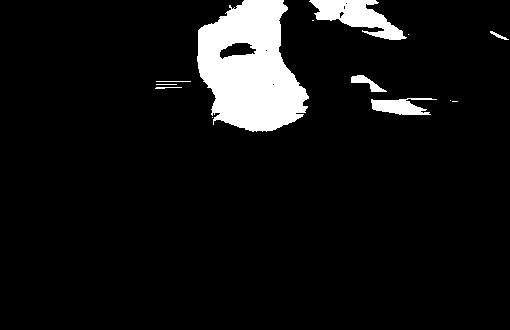##### 检测轮廓##### 图像膨胀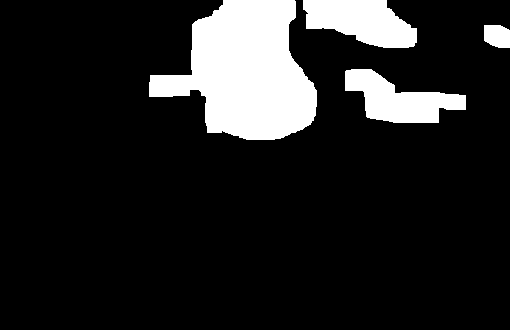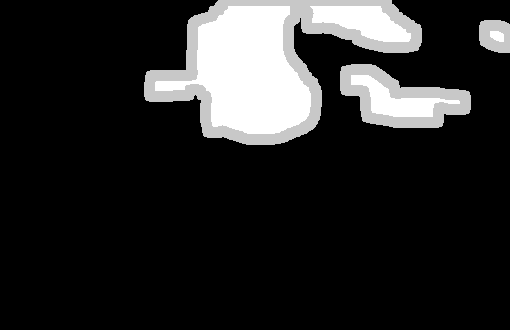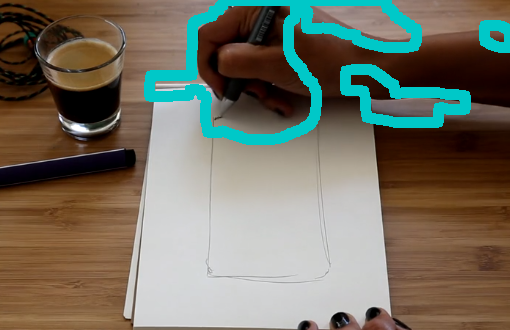#### 用OpenCV和Python构建车辆检测系统

##### 导入库
import os
import re
import cv2 # opencv library
import numpy as np
from os.path import isfile, join
import matplotlib.pyplot as plt
##### 导入视频帧

# get file names of the frames
col_frames = os.listdir('frames/')

# sort file names
col_frames.sort(key=lambda f: int(re.sub('\D', '', f)))

# empty list to store the frames
col_images=[]

for i in col_frames:
# append the frames to the list
col_images.append(img)
##### 数据探索

# plot 13th frame
i = 13

for frame in [i, i+1]:
plt.imshow(cv2.cvtColor(col_images[frame], cv2.COLOR_BGR2RGB))
plt.title("frame: "+str(frame))
plt.show()# convert the frames to grayscale
grayA = cv2.cvtColor(col_images[i], cv2.COLOR_BGR2GRAY)
grayB = cv2.cvtColor(col_images[i+1], cv2.COLOR_BGR2GRAY)

# plot the image after frame differencing
plt.imshow(cv2.absdiff(grayB, grayA), cmap = 'gray')
plt.show()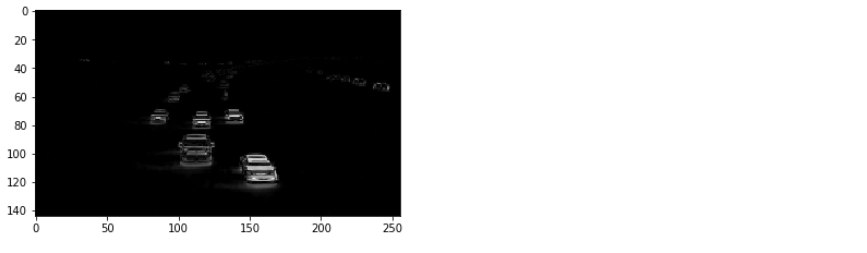##### 图像预处理

diff_image = cv2.absdiff(grayB, grayA)

# perform image thresholding
ret, thresh = cv2.threshold(diff_image, 30, 255, cv2.THRESH_BINARY)

# plot image after thresholding
plt.imshow(thresh, cmap = 'gray')
plt.show()# apply image dilation
kernel = np.ones((3,3),np.uint8)
dilated = cv2.dilate(thresh,kernel,iterations = 1)

# plot dilated image
plt.imshow(dilated, cmap = 'gray')
plt.show()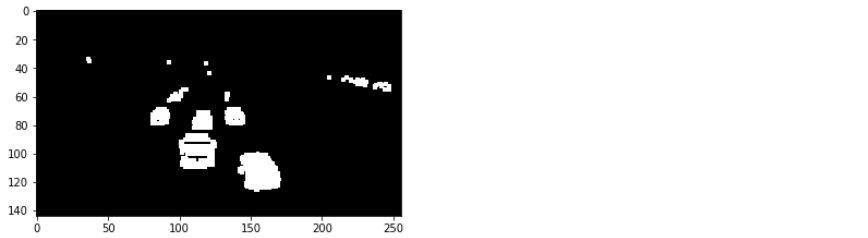# plot vehicle detection zone
plt.imshow(dilated)
cv2.line(dilated, (0, 80),(256,80),(100, 0, 0))
plt.show()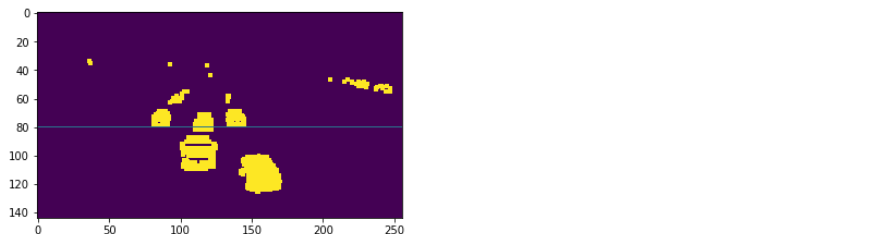# find contours
contours, hierarchy = cv2.findContours(thresh.copy(),cv2.RETR_TREE,cv2.CHAIN_APPROX_NONE)

valid_cntrs = []

for i,cntr in enumerate(contours):
x,y,w,h = cv2.boundingRect(cntr)
if (x <= 200) & (y >= 80) & (cv2.contourArea(cntr) >= 25):
valid_cntrs.append(cntr)

# count of discovered contours
len(valid_cntrs)

dmy = col_images.copy()

cv2.drawContours(dmy, valid_cntrs, -1, (127,200,0), 2)
cv2.line(dmy, (0, 80),(256,80),(100, 255, 255))
plt.imshow(dmy)
plt.show()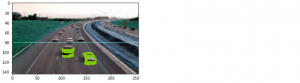##### 视频中的车辆检测

1. 对每对连续帧应用帧差分

2. 对上一步的输出图像应用图像阈值

3. 对上一步的输出图像进行图像放大

4. 在上一步的输出图像中查找轮廓

5. 检测区域出现的候选轮廓

6. 保存帧与最终轮廓

# kernel for image dilation
kernel = np.ones((4,4),np.uint8)

# font style
font = cv2.FONT_HERSHEY_SIMPLEX

# directory to save the ouput frames
pathIn = "contour_frames_3/"

for i in range(len(col_images)-1):

# frame differencing
grayA = cv2.cvtColor(col_images[i], cv2.COLOR_BGR2GRAY)
grayB = cv2.cvtColor(col_images[i+1], cv2.COLOR_BGR2GRAY)
diff_image = cv2.absdiff(grayB, grayA)

# image thresholding
ret, thresh = cv2.threshold(diff_image, 30, 255, cv2.THRESH_BINARY)

# image dilation
dilated = cv2.dilate(thresh,kernel,iterations = 1)

# find contours
contours, hierarchy = cv2.findContours(dilated.copy(), cv2.RETR_TREE,cv2.CHAIN_APPROX_NONE)

# shortlist contours appearing in the detection zone
valid_cntrs = []
for cntr in contours:
x,y,w,h = cv2.boundingRect(cntr)
if (x <= 200) & (y >= 80) & (cv2.contourArea(cntr) >= 25):
if (y >= 90) & (cv2.contourArea(cntr) < 40):
break
valid_cntrs.append(cntr)

# add contours to original frames
dmy = col_images[i].copy()
cv2.drawContours(dmy, valid_cntrs, -1, (127,200,0), 2)

cv2.putText(dmy, "vehicles detected: " + str(len(valid_cntrs)), (55, 15), font, 0.6, (0, 180, 0), 2)
cv2.line(dmy, (0, 80),(256,80),(100, 255, 255))
cv2.imwrite(pathIn+str(i)+'.png',dmy)  
##### 准备视频

# specify video name
pathOut = 'vehicle_detection_v3.mp4'

# specify frames per second
fps = 14.0

frame_array = []
files = [f for f in os.listdir(pathIn) if isfile(join(pathIn, f))]
files.sort(key=lambda f: int(re.sub('\D', '', f)))
for i in range(len(files)):
filename=pathIn + files[i]

height, width, layers = img.shape
size = (width,height)

#inserting the frames into an image array
frame_array.append(img)


out = cv2.VideoWriter(pathOut,cv2.VideoWriter_fourcc(*'DIVX'), fps, size)

for i in range(len(frame_array)):
# writing to a image array
out.write(frame_array[i])

out.release()

### 尾注

http://panchuang.net/

sklearn机器学习中文官方文档：
http://sklearn123.com/

http://docs.panchuang.net/